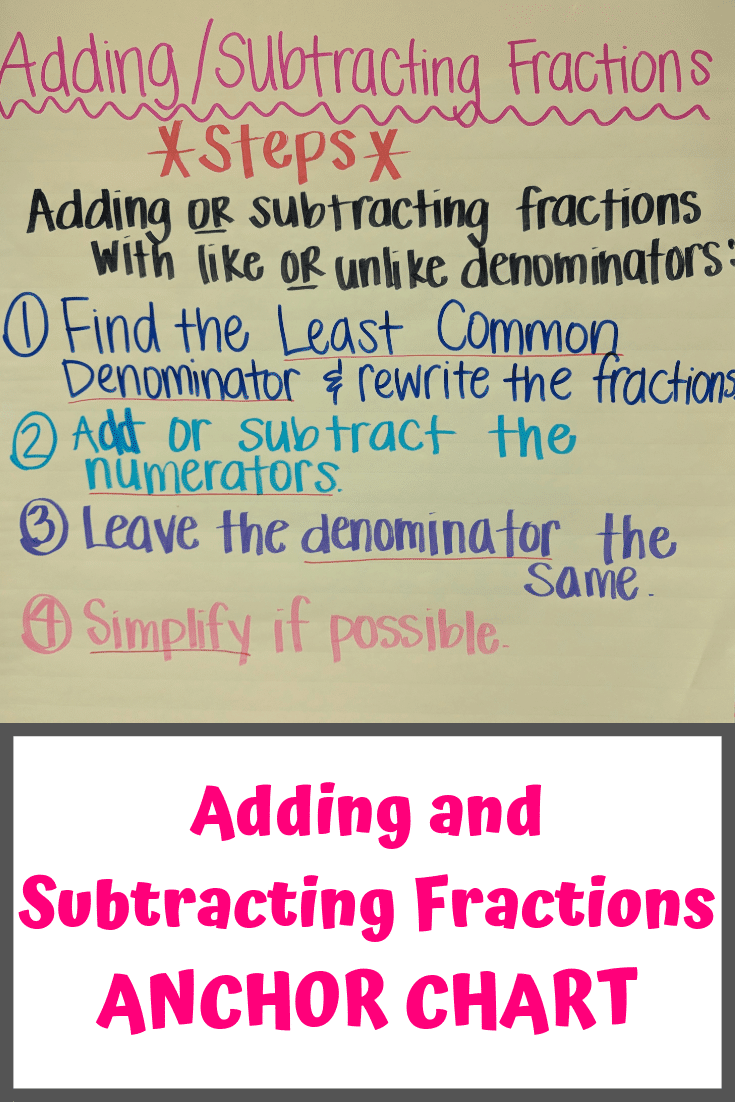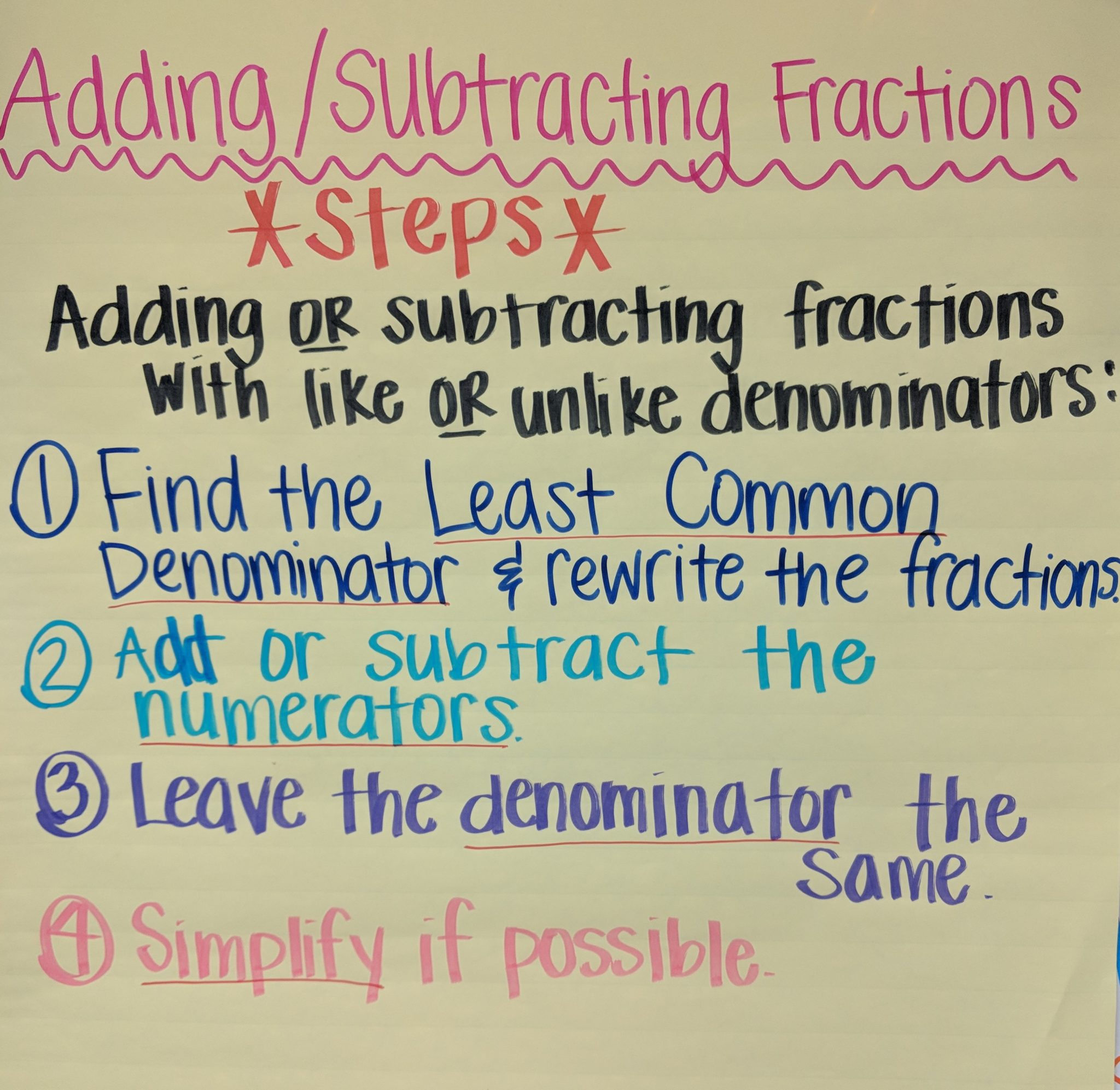# Adding Fractions Anchor Chart for Elementary Math Classroom

One visual teaching tool I like to have in my elementary school math classroom is this anchor chart for fractions. It helps students master the process of adding and subtracting fractions, which can be a difficult concept for some students.  Mastering this building block concept is important, because teaching more complicated math involving fractions rests on understanding basic fraction operations.

For some reason, when my students see fractions or decimals they just freak out. (I have made so many anchor charts about these two concepts. For instance, my rounding decimals anchor chart and multiplying decimals anchor chart. ) And unlike denominators on the fractions they are working with make them even more anxious.## Creating an Adding Fractions Anchor Chart

Creating this anchor chart with my class helps them master the concept of adding and subtracting fractions.

I find it most effective to create the anchor chart along with my students. If the first chart turns out messy, I recreate it when the students are home for the day. Going through the process with them though helps them visualize and learn better.

As we are creating the anchor chart, we do a few examples of adding and subtracting fractions so they can go through the entire process.  I use examples where the denominators are the same and different.   After they understand the process of adding or subtracting two fractions, I’ll give them three or four fractions to see if they can extrapolate the concept further.  I also don’t make it to where finding the least common denominator of multiple fractions is too difficult.

Also, even though you have an anchor chart, it’s still important to teach the “why” of how fraction addition and subtraction works first (or at least while creating the visual.)

## Fraction Anchor Chart Steps and Details

First, it simplifies the process for students if they realize the same rules apply for both adding and subtracting fractions.  No need to remember two different methods for these operations.  I thought about doing two separate charts (one adding fractions anchor chart and one subtracting fractions anchor chart.) But since the processes are so similar, I combined them into one anchor chart covering both concepts.

Second, these steps apply whether the denominators are like or unlike.   Also, to help them remember the difference between denominators and numerators, I tell them the denominators are “down” or on the bottom.

### Here are the steps:

1. First, find the least common denominator. I have another anchor chart around my classroom that reminds them how to find a least common denominator. Once this is done, rewrite the fractions using the new least common denominator.
2. Add or subtract the numerators of the rewritten fractions.
3. Leave the denominator the same at this point.
4. Last, simplify, if possible. For some reason, simplifying fractions seems to be easier for my students to understand than the concept of adding and subtracting fractions.These steps allowed my students to master how to add and subtract fractions.  So far, I’ve felt like the anchor chart has provided a great visual reminder for my students.  When the chart comes down for state testing, I often find them staring at the place on the wall where the chart was while reminding themselves of the steps.

This anchor chart is a part of my must-have math anchor charts for my third and fifth grade math classrooms.  These have been a great teaching tool for my students!